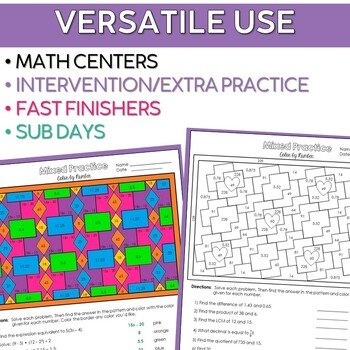# 5th-6th Grade Math Color by Number Activities Mixed Math Review

Rated 4.6 out of 5, based on 5 reviews
5 Ratings;
5th - 7th, Homeschool
Subjects
Resource Type
Standards
Formats Included
• PDF
Pages
25 pages
\$5.50
List Price:
\$6.00
You Save:
\$0.50
\$5.50
List Price:
\$6.00
You Save:
\$0.50
Report this resource to TPT
##### Also included in
1. This custom math bundle is 20% off and includes color by number and problem solving resourcesResources in this bundle:6th Grade Math Color by Number Activities Bundle7th Grade Math Color by Number Activities Bundle 5th-6th Grade Math Color by Number Activities Mixed Math Review**********************
Price \$27.50Original Price \$43.00Save \$15.50
2. Get your emergency sub plans set with this variety of activities that will keep students engaged when you need to be out.Use these no-prep resources for ANY day you need to be out of the classroom!Many of the concepts covered in these activities could be review for your students, which can be perfec
Price \$15.00Original Price \$20.50Save \$5.50

### Description

This set of 12 mixed math review color by number activities is great for fast finishers, math centers, review homework, or sub plans – so many uses!

• Six of the color by numbers have a seasonal design, while six are geometric patterns.

This easy-to-use resource for 5th-6th grade math includes:

• 12 color by number sheets

Each sheet has 10 questions, which are a mix of concepts (generally 5th-6th grade, but great for upper grade review as well).

Questions require students to:

• Multiply and divide whole numbers
• Complete all decimal operations
• Complete all fraction operations
• Find LCM, GCF
• Solve one-step equations
• Convert fractions to decimals and decimals to fractions
• Solve proportions
• Evaluate exponential expressions
• Use order of operations
• Convert percent to decimal and fraction
• Find percent of a number
• Compare decimals
• Find mean, median, range
• Find absolute value
• Find unit rates and ratios
• Find area of square
• Find equivalent expressions
• Find cube roots

**Some of these concepts are included more frequently(fraction and decimal concepts), while others are only used a few times (unit rates, absolute value, area, cue roots).

⭐️⭐️⭐️⭐️⭐️"Students love to color, I get more participation with these sheets."

⭐️⭐️⭐️⭐️ "This was a great "I'm done, now what?" activity for my students to do after they finished their work. Thanks!"

⭐️⭐️⭐️⭐️⭐️"This resource was perfect for my 6th grade math students! Thank you!"

Related Resources:

New Year's Mixed Math Practice 6th Grade Color by Number

New Year's Mixed Math Practice 7th Grade Color by Number

Valentine's Day Mixed Math Practice Activities

Fall Mixed Math Practice Color by Number

Fall Mixed Math Practice Footloose Task Cards

Holiday Mixed Math Practice Color by Number

Winter Mixed Math Review Color by Number

Winter Math Review Footloose Task Cards

You might also like:

5th Grade Color by Number Mini-Bundle

Problem Solving Sets

********************************************************************

Please keep in touch by following me, to be notified when new products are uploaded! Resources are 1/2 off for the first 24 hours, so it pays to follow:-)

********************************************************************

Connect with Me:

Check out my Blog

*********************************************************************

Permission to copy for single classroom use only.

Total Pages
25 pages
Included
Teaching Duration
N/A
Report this resource to TPT
Reported resources will be reviewed by our team. Report this resource to let us know if this resource violates TPT’s content guidelines.

### Standards

to see state-specific standards (only available in the US).
Add and subtract fractions with unlike denominators (including mixed numbers) by replacing given fractions with equivalent fractions in such a way as to produce an equivalent sum or difference of fractions with like denominators. For example, 2/3 + 5/4 = 8/12 + 15/12 = 23/12. (In general, 𝘢/𝘣 + 𝘤/𝘥 = (𝘢𝘥 + 𝘣𝘤)/𝘣𝘥.)
Apply and extend previous understandings of multiplication to multiply a fraction or whole number by a fraction.
Apply and extend previous understandings of division to divide unit fractions by whole numbers and whole numbers by unit fractions.
Interpret and compute quotients of fractions, and solve word problems involving division of fractions by fractions, e.g., by using visual fraction models and equations to represent the problem. For example, create a story context for (2/3) ÷ (3/4) and use a visual fraction model to show the quotient; use the relationship between multiplication and division to explain that (2/3) ÷ (3/4) = 8/9 because 3/4 of 8/9 is 2/3. (In general, (𝘢/𝘣) ÷ (𝘤/𝘥) = 𝘢𝘥/𝘣𝘤.) How much chocolate will each person get if 3 people share 1/2 lb of chocolate equally? How many 3/4-cup servings are in 2/3 of a cup of yogurt? How wide is a rectangular strip of land with length 3/4 mi and area 1/2 square mi?
Fluently divide multi-digit numbers using the standard algorithm.